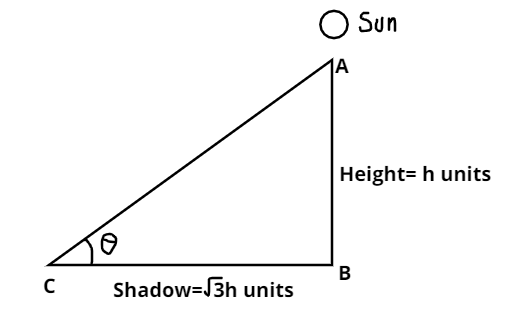Courses
Courses for Kids
Free study material
Offline Centres
MoreLast updated date: 07th Dec 2023
Total views: 384.9k
Views today: 3.84k

# The angle of elevation of the sun when the length of the shadow of pole is $\sqrt 3$times the height of the pole is$(A){\text{ 3}}{{\text{0}}^0} \\ (B){\text{ 4}}{{\text{5}}^0} \\ (C){\text{ 6}}{{\text{0}}^0} \\ (D){\text{ 1}}{{\text{5}}^0} \\$Verified
384.9k+ views
Hint: Draw figure and then use trigonometry identity $\cot \theta = \dfrac{{Base}}{{Perpendicular}}$.Let AB be the height of the pole and BC be its shadow.
As, we are given the ratio of the length of the pole and its shadow.
Let the height of the pole be AB$= h$ units.
So, the length of its shadow will be BC $= \sqrt 3 h$ units.
So, we must find an angle of elevation.
Now, we can only use the given condition.
So, the best way to find the angle of elevation using trigonometric formulas.
So, solving using given conditions.
Let, $\angle ACB = \theta$.
And , $\theta$ will be the angle of elevation of sun
And we know that $\cot \theta = \dfrac{{Base}}{{Perpendicular}}$.
Here, the base will be BC.
And, perpendicular will be AB.
So, here putting the value of base and perpendicular in $\cot \theta$ we will get.
$\cot \theta = \dfrac{{BC}}{{AB}} = \dfrac{{\sqrt 3 h}}{h} = \sqrt 3$
So, from the above equation we can write,
$\theta = {\cot ^{ - 1}}\left( {\sqrt 3 } \right)$ (1)
Using inverse trigonometric formulas. We can say that $\theta = {30^0}$
Hence, the angle of elevation of the sun will be ${30^0}$.
Hence, the correct Option will be A.

Note: Whenever we come up with these types of problems, first we should draw the figure according to the given conditions in question, then we will use the trigonometric functions to get the angle of elevation which will be the easiest and efficient method. And we should remember that object and its shadow are always perpendicular to each other.